Function Repository Resource:

# ColumnSpaceBasis

Return a basis for the subspace spanned by the columns of a matrix

Contributed by: Dennis M Schneider
 ResourceFunction["ColumnSpaceBasis"][mat] returns a basis for the column space of mat.

## Details and Options

The basis returned by ResourceFunction["ColumnSpaceBasis"][mat] is comprised of the pivot columns of mat.

## Examples

### Basic Examples (2)

Define a matrix:

 In:=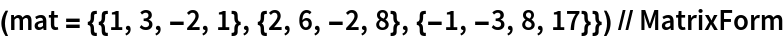Out=The first and third columns of the matrix are pivot columns:

 In:=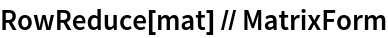Out=Therefore, the first and third columns of the matrix form a basis for the column space:

 In:=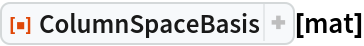Out=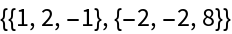Define a complex matrix:

 In:=Out=The first three columns are all pivot columns:

 In:=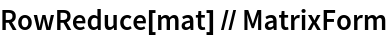Out=Therefore, the first three columns of the matrix form a basis for its column space:

 In:=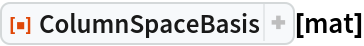Out=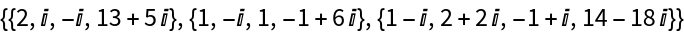## Publisher

Dennis M Schneider

## Version History

• 1.0.0 – 08 August 2019